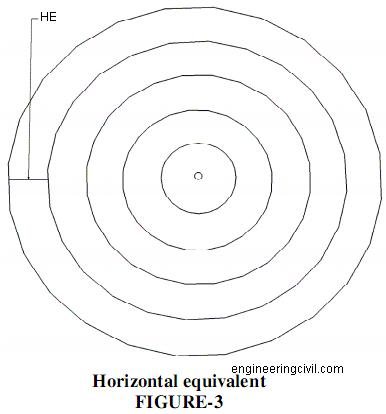Search

# Explain Terms Used in Contouring

Posted in Surveying |Email This Post |

Define Contour Line
A Contour line is an imaginary outline of the terrain obtained by joining its points of equal elevation. In our example of the cone, each circle is a contour line joining points of same level.

Define Contour Interval (CI)
Contour interval is the difference between the levels of consecutive contour lines on a map. The contour interval is a constant in a given map. In our example, the contour interval is 1m.

Define Horizontal Equivalent (HE)
Horizontal equivalent is the horizontal distance between two consecutive contour lines measured to the scale of the map.Gradient represents the ascending or descending slope of the terrain between two consecutive contour lines. The slope or gradient is usually stated in the format 1 in S, where 1 represents the vertical component of the slope and S its corresponding horizontal component measured in the same unit.

The gradient between two consecutive contour lines can also be expressed in terms of Tan Q(theta) as follows:
Tan Q (theta) = CI / HE … both measured in the same unit.

We at engineeringcivil.com are thankful to Mr Ramasesh Iyer for submitting this useful information to us.

More Entries :